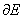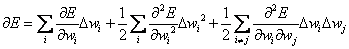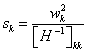Home News About Me Ph. D. Thesis Abstract Table of Contents 1. Introduction 2. Theory  Fundamentals of the Multivariate Data Analysis 2.1. Overview of the Multivariate Quantitative Data Analysis 2.2. Experimental Design 2.3. Data Preprocessing 2.4. Data Splitting and Validation 2.5. Calibration of Linear Relationships 2.6. Calibration of Nonlinear Relationships 2.7. Neural Networks  Universal Calibration Tools 2.8. Too Much Information Deteriorates Calibration 2.8.1. Overfitting, Underfitting and Model Complexity 2.8.2. Neural Networks and the Complexity Problem 2.8.3. Brute Force Variable Selection 2.8.4. Variable Selection by Stepwise Algorithms 2.8.5. Variable Selection by Genetic Algorithms 2.8.6. Variable Selection by Simulated Annealing 2.8.7. Variable Compression by Principal Component Analysis 2.8.8. Topology Optimization by Pruning Algorithms 2.8.9. Topology Optimization by Genetic Algorithms 2.8.10. Topology Optimization by Growing Neural Network Algorithms 2.9. Measures of Error and Validation 3. Theory  Quantification of the Refrigerants R22 and R134a: Part I 4. Experiments, Setups and Data Sets 5. Results  Kinetic Measurements 6. Results  Multivariate Calibrations 7. Results  Genetic Algorithm Framework 8. Results  Growing Neural Network Framework 9. Results  All Data Sets 10. Results  Various Aspects of the Frameworks and Measurements 11. Summary and Outlook 12. References 13. Acknowledgements Publications Research Tutorials Downloads and Links Contact Search Site Map Print this Page2.8.8.   Topology Optimization by Pruning Algorithms

The oldest and very popular approach to topology optimization of neural networks is based on destructive pruning algorithms ,,-. These algorithms start with a trained fully connected neural network, remove certain network elements and then retrain the network. The steps of removing and retraining are repeated until a certain stopping criterion is fulfilled. Besides of the algorithm "Skeletonization" , only links are considered as candidates to be removed. Neurons are automatically removed, if all corresponding input or output links have been removed. In this work the two pruning algorithms "Optimal Brain Surgeon" and "Magnitude Based Pruning" are used, which were identified as most promising pruning methods for sensor data in a comparison . A combination of both algorithms can be found in .

Magnitude Based Pruning
The Magnitude Based Pruning (MP) is the simplest approach among the pruning algorithms, which removes the links with the smallest weights. This approach originates in the linear regression, as the variables with the smallest regression coefficients of a linear regression are least important. Yet, Despagne et al. demonstrated that for data sets with both, linear and nonlinear relationships, this assumption is not valid as primarily links of linear variables are removed . On the other hand, it was shown in , that this simple and very fast algorithm performs as well as the other algorithms as long as not too many links are removed.

Optimal Brain Surgeon
The Optimal Brain Surgeon (OBS) algorithm  estimates the increase of the training error when a link is deleted. Then the links with the smallest increase, which is also called saliency, are deleted. To determine the saliencies, the changes of the cost functionassociated with a perturbation of the weight matrix W has to be evaluated. The termis approximated by a second-order Taylor series expansion:(20)

As the training is stopped when the neural network has converged to a minimum, the first term can be considered as being zero. The second and third term are the so-called Hessian Matrix H, whereby the Optimal Brain Damage algorithm  neglects the third term resulting in faster yet less reliable pruning results . The saliency of a weight k is then calculated as(21)

Discussion
Although the pruning algorithms have been applied in several fields of chemistry and analytics ,,, the general application of these algorithms is controversially discussed in literature. In congruence with the theory that pruned sparse neural networks should generalize better, improved predictions were found in references ,, whereas a deterioration of the prediction ability was found in references ,. Another problem of the pruning algorithms is the need of a reference network for the removal of the network elements. This reference network has to be designed by the user and the final optimal network found by the algorithm cannot be more complex than this reference network. This means that the "solution" is biased by the choice of the user for the reference network as the pruning algorithms are highly sensitive to local minima of the error surface ending up in completely different network topologies and different variables selected for different reference networks.

 Page 55 © Frank Dieterle, 03.03.2019